Practise Maths Subtraction

R115.00 Price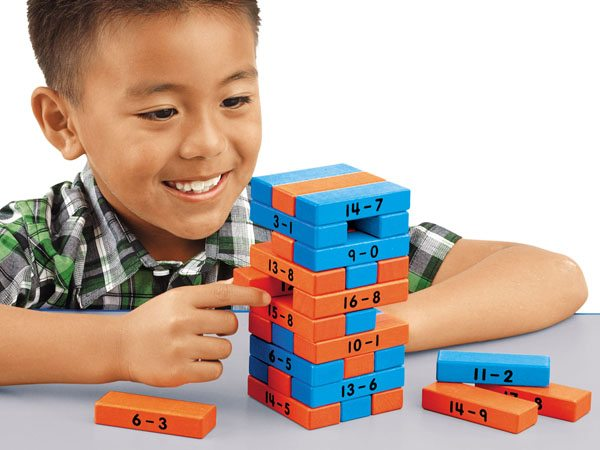Subtraction Tower Of Math Game

R914.25 PriceR150.00 Price

Learning Maths Beginner Abacus

R300.00 PriceR800.00 Price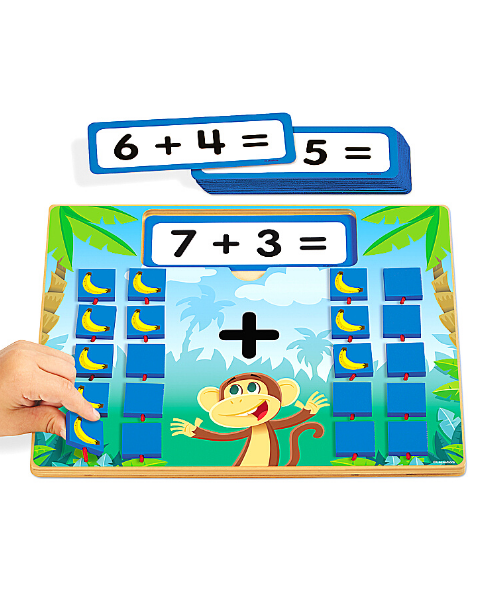Flip And Solve Maths Boards – Addition

R850.00 Price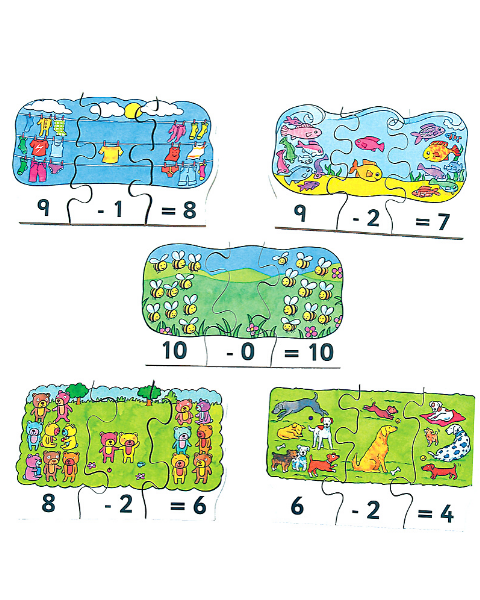Sum Fun Subtraction

R630.00 PriceR630.00 Price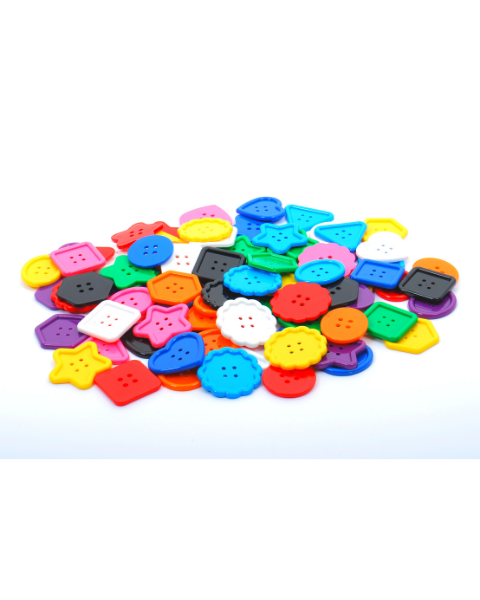Assorted Large Buttons

R450.00 Price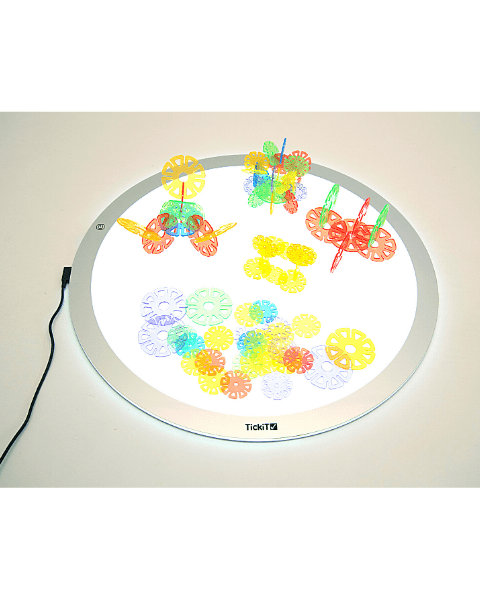Round Light Panel

R5,600.00 Price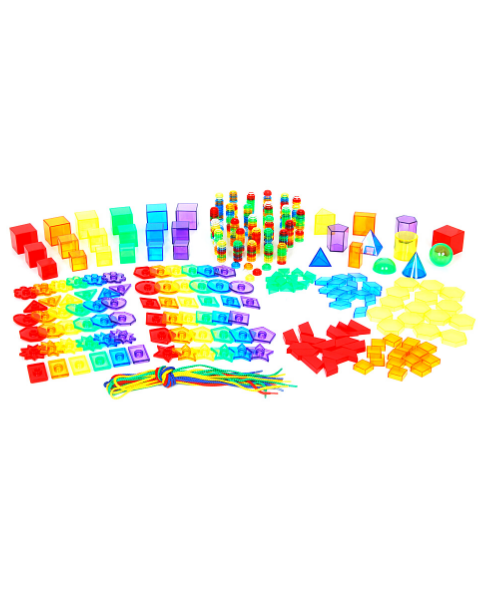Early years Maths Resource Kit

R2,165.00 Price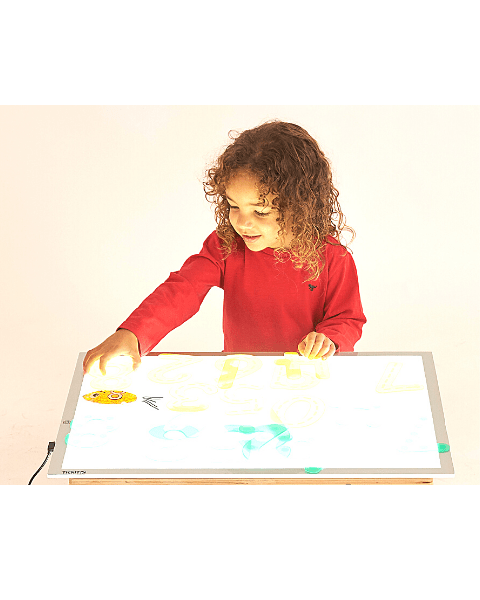A2 Light Panel

R3,875.00 Price

Showing 1 to 12 of 69 (6 Pages)